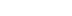/

# Physics on the Back of a Cocktail Napkin

Pool, like many sports, is dominated by the laws of physics. Good players have an excellent sense of the application of force, the physics of collisions, and the influence of friction on objects in motion. Jeff Lander uses pool to demonstrate how a solid physical foundation can actually create interesting game play, as well as providing some ideas that can be converted easily to other sports such as golf or tennis.

I am generally pretty disciplined about working normal hours during the week. However, on Fridays I like to shoot pool and eat pizza at the local sports bar. Since happy hour starts at three, I sometimes need to move work down the street. I was working out how to win a serious game of nine ball when one of those geeky discussions broke out about pool table physics. Pool, like many sports, is dominated by the laws of physics. Good players have an excellent sense of the application of force, the physics of collisions, and the influence of friction on objects in motion.

Last month I described how friction could be used to increase the realism of the physics model in real-time games. The demo program made it possible to see how various coefficients affected a mass-and-spring model. However, it wasn't very much fun. In order to demonstrate how a solid physical foundation can actually create interesting game play, I need to pull some of these concepts together into a real application. A pool table simulation is a natural choice. It will allow me to apply many of the techniques I have covered as well as provide some ideas that can be converted easily to other sports such as golf or tennis.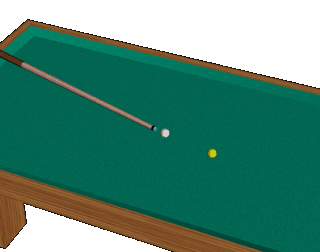Like most things in life, pool provides an excellent venue for a physics lesson.

Two Ball, Corner Pocket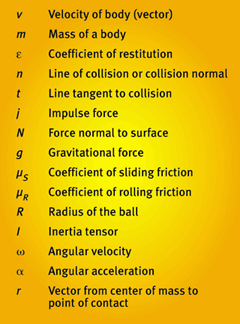Table 1. A summary of the notation used in this article.

In order to understand pool, I need to understand collisions between billiard balls. Fortunately, billiard balls are all spheres of equal size and weight. That makes the collision calculations a bit easier. Let me begin by looking at the frictionless case. I suspect that if I ignore the ball's rotation and do not consider friction, the ball collision will behave exactly like a particle at the ball's center of mass. That would be great, as I could use the code from a previous column. However, I want to make sure. Figure 1 shows a typical collision.

Ball A is moving at a speed of 20 feet per second and collides with ball B at a 40 degree angle. In order to determine the velocity of each ball after the collision, I need to apply dynamics. A collision between two rigid bodies, which occurs in a very short time and during which the two bodies exert relatively large forces on each other, is called an impact. The force between these two bodies during the collision is called an impulsive force, which is symbolized by j. The common normal to the surfaces of the bodies in contact is called the line of collision, represented as n in Figure 1.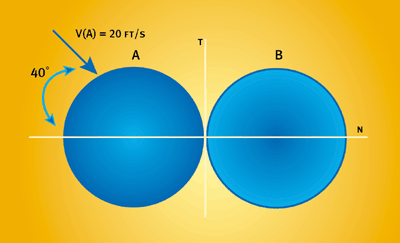Figure 1. A simple collision between two billiard balls.

The first step is to break the initial velocity of ball A into its components along the line of collision, n, and the tangent to the collision, t.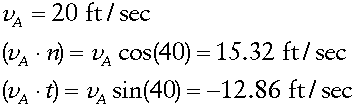The impulsive force acting during the collision is directed along the line of collision. Therefore, the t component of the velocity of each ball is not changed.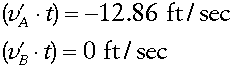In order to determine the new velocity along the line of collision, I need to look at the impulsive force between the bodies. The impulse acts on both bodies at the same time. You may remember Newton's third law of motion, the forces exerted by two particles on each other are equal in magnitude and opposite in direction.

Since the impulse forces are equal and opposite, momentum is therefore conserved before and after the collision. Remember that the momentum of a rigid body is mass times velocity (mv).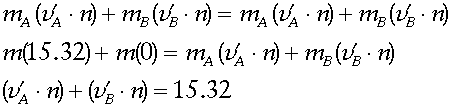(Eq. 1)

This equation can't be solved without some more information. In my previous article "Collision Response: Bouncy, Trouncy, Fun", I discussed the coefficient of restitution. This is the scalar value between 0 and 1 relating the velocities of bodies before and after a collision via the formula: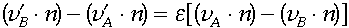(Eq. 2)

For this example, I'm using a coefficient of restitution e of 0.8. I can use this formula to create a second equation.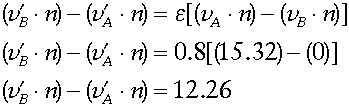(Eq. 3)

Solving Equations 1 and 3, I get the velocities of the two billiard balls after the collision.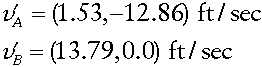In order to solve this problem in the simulation, I need to derive the impulse force directly. The impulse force creates a change in momentum of the two bodies with the following relationship.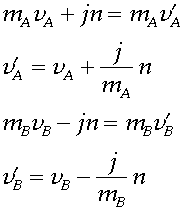(Eq. 4)

These formulas can be combined with Equation 2 to determine the impulse force given the relative velocity and the coefficient of restitution.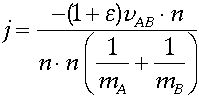(Eq. 5)

You can plug Equation 5 back into the example problem and make sure it works. Remember that because of Newton's third law, the impulse is equal and opposite for the two colliding bodies. When you apply Equation 5 to the B ball, remember to negate it.

Those of you who read Chris Hecker's column on collision response ("Physics, Part 3: Collision Response," Behind the Screen, Game Developer, February/March 1997) will recognize Equation 4 as the impulse equation for a general body that does not rotate. When we do not consider the rotation of the billiard balls, they behave exactly like the particles used in my March 1999 mass-and-spring demo. My suspicion was correct, and I can use the particle dynamics system as a base for the demo.

For many applications, this would probably be more than enough to get a decent physical simulation. In fact, I imagine many pool simulations end right there. This level of simulation is probably sufficient for other games, such as pinball. However, anyone who has played much pool knows that this is not the end of the story. The rotation of the ball caused by the reaction with the table makes a tremendous difference in the realism of the simulation.

Slip Sliding Away

When a billiard ball is hit with the cue stick, the ball starts moving across the table. If the ball is struck along its center of mass, the ball is not initially rotating.

However, soon the ball starts rolling along. Friction between the ball and the table causes this roll to occur. You can see this situation in Figure 2.

The ball is traveling with the forward velocity, v. In my article "The Trials and Tribulations of Tribology" , I discussed the use of kinetic friction via the Coulomb dry friction model. For our purposes, I'm going to call this force "sliding friction." The force of friction applied to a body sliding over a surface is given by the following formula: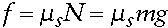(Eq. 6)

The friction force is applied in the direction opposite the velocity. Since this force is applied to the surface of the ball and not its center of mass, the frictional force causes angular acceleration in the ball. As the ball rolls across the table, the angular velocity increases because of this sliding friction force. This continues until a time of equilibrium is reached, where the velocity of the point contacting the table equals the velocity of the center of mass. At this time, the ball is no longer sliding and is now rolling on the table. This situation is called a natural roll or rolling without sliding. In mathematical terms, this situation happens when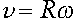(Eq. 7)

where v is the velocity of the ball, w (LUKE-this is a lowercase omega) is the angular velocity of the ball, and R is the ball's radius.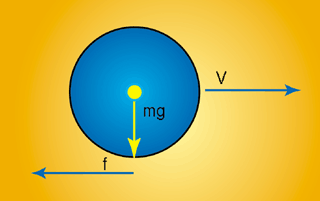Figure 2. A billiard ball sliding across the table.

Now I need to show how the angular acceleration actually changes. This is going to mean bringing up another term, the inertia tensor, or I. You may remember from Chris Hecker's column on 3D physics ("Physics, Part 4: The Third Dimension," Behind the Screen, June 1997) that the inertia tensor relates the angular velocity of a body to the angular momentum of that body. For arbitrarily complex objects, creating the inertia tensor can be quite difficult. However, for a uniform sphere where the density is uniform across the sphere, it's quite easy. The inertia tensor for a sphere is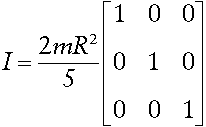(Eq. 8)

Therefore, the product of this matrix with any vector is a simple scaling of that vector. The relationship between the angular acceleration and the friction force then becomes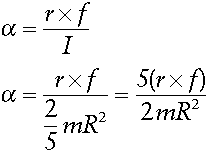(Eq. 9)

If I now take a look at the problem in Figure 2, I can calculate how long it will take for the ball to achieve natural roll given an initial velocity v. From the principle of impulse and momentum, I know some information about the linear momentum and angular velocity of the ball at a later time.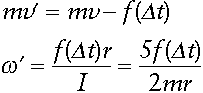In other words, the momentum at some later time is the initial momentum minus the impulse created by the friction force, f. I know the friction force from Equation 6.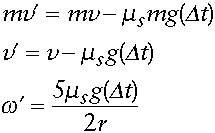At the point of natural roll, I know the state of equilibrium between angular and linear velocity from Equation 7.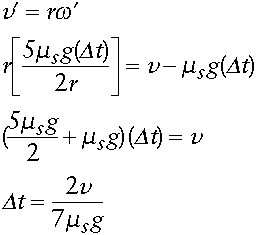So you can see that as a result of the friction force of the table, a sliding billiard ball will always reach a point where it is rolling without sliding on the table. This is the type of realism I want to have in the simulation. A ball when struck should slide across the table, slowly settling to a state where it is rolling without slipping.

How Do I Stop This Crazy Thing?

One glaring problem remains. I can run the simulation with all of the physics discussed so far. When struck hard, a billiard ball will slide and then roll. Once the ball has reached this natural roll, there is nothing in my simulation that will keep it from continuing to roll forever. The friction force is gone since the point of contact is not moving relative to the table. I need to add another force that will slow down a rolling ball. I can add another frictional force, called rolling friction, which is applied when the ball is in natural roll. The form of rolling friction is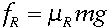It is applied exactly like the sliding friction whenever the natural roll conditions apply. It is important to note that the coefficients of rolling and sliding friction are not necessarily the same. Think of a ball moving on a rubber surface. The coefficient of sliding friction would be very high. However, the rolling friction would be comparatively low, allowing the ball to roll across the surface easily.

Bumpin' the Cush

Collision with the table's side cushions can be handled in a couple of ways. If I consider the table to be completely 3D, I will need to handle 3D collision between the ball and the cushion. That would be the most realistic. It would allow the balls to move up and down as well as side to side. This might be interesting if I wanted to be able to perform a jump shot (when the cue ball jumps up and over other balls on the table). However, I'm not really ready to tackle the physical and interface issues involved in making this happen.

If I'm willing to give up the flexibility of allowing the balls to move in 3D, things become a bit easier. For one thing, I can eliminate the gravitational force acting on the ball. A ball sitting on a table is in a constant collision battle with the table top. By getting rid of the gravitational force, I save having to deal with the ball constantly interpenetrating the table. I still need to keep track of the gravitational force as it is used in calculating the friction force applied by the table. However, I just assume the balls are in constant sliding or rolling contact with the table.

Also, if I ignore vertical motion of the balls, I can turn the collision with the side cushions into a 2D computational geometry problem. The boundaries of the table are now line segments and I can use the 2D collision detection routines developed in my article "Crashing into the New Year".

Later, I may wish to allow the balls to jump. It would then be easy enough to convert the collision back to 3D. These kinds of decisions are made all the time during the game production process. Since game simulation is all about speed versus realism, simplifying the problem if it works for your particular application makes sense.

Rack 'Em Up

Using these techniques, I have created a demonstration of a simple pool table. The simulation uses rolling and sliding friction to simulate the way a real billiard ball moves across a table. Collision between balls is handled through conservation of momentum and the elastic collision model. There are several areas that still need work. Of course, I didn't talk about applying "English" to the shot by striking the ball off the center of mass. This technique is what makes shots such as a Masse, draw, or topspin possible. This is largely just a matter of where the impulse from the cue stick is applied. Also, the lack of friction between colliding balls does not allow effects such as collision-induced spin.

Another problem arises when we consider simultaneous collision between several billiard balls. When calculating the resulting force when two balls collide, it was fairly easy to determine the resulting force. However, when several balls collide simultaneously, the law of conservation of momentum becomes much harder to enforce. In order to calculate the resultant forces correctly, I need to solve several simultaneous equations. Obviously, this tends to complicate things quite a bit.

Alas, that will have to wait for another time. Until then, see if you can modify the source code to handle these effects. You can download the source code and the executable application off the Game Developer web site at http://www.gdmag.com.

For Further Info:

Beer, Ferdinand, and E. Russell Johnston. Vector Mechanics for Engineers: Statics and Dynamics, Sixth Ed. New York: WCB/McGraw-Hill, 1997.

Hecker, Chris. "Behind the Screen," Game Developer (October/November 1996-June 1997). Also available on Chris's web site, http://www.d6.com.

When not wasting time at the pub eating hot wings and shooting pool, Jeff can be found at Darwin 3D. There he creates real-time 3D graphics for a variety of applications. Drop him a line at [email protected].

### Latest Jobs

#### Infinity Ward

Woodland Hills, California
11.3.21
Sr. Multiplayer Design Scripter/Programmer

#### Disbelief

Cambridge, Massachusetts
11.3.21
Jr. Programmer

#### 4A Games

Malta
11.3.21
Lead Game Systems Designer New IP

#### XSEED

Torrance, California
11.3.21
More Jobs